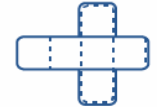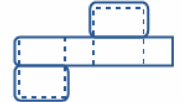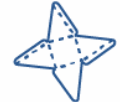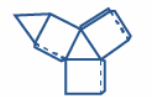Identify the nets given below and match the name of the corresponding solid from the column provided.Matrix:COLUMN I(Net)COLUMN II(Name of Solid)(a) (i) Square pyramid(b) (i) Square pyramid(b) (ii) Cone(c)  (ii) Cone(c)  (iii) Triangular prism(d) (iii) Triangular prism(d) (iv) Cuboid(e) (iv) Cuboid(e) (v) Cube(f) (v) Cube(f) (vi) cylinder

# Identify the nets given below and match the name of the corresponding solid from the column provided.Matrix:COLUMN I(Net)COLUMN II(Name of Solid)(a)(i) Square pyramid(b)(i) Square pyramid(b) (ii) Cone(c)(ii) Cone(c)  (iii) Triangular prism(d)(iii) Triangular prism(d) (iv) Cuboid(e)(iv) Cuboid(e) (v) Cube(f)(v) Cube(f) (vi) cylinder

1. A
a-v; b-iv; c-ii; d-vi; e-i; f-iii
2. B
a-vi; b-v; c-iii; d-iv; e-i; f-iii
3. C
a-v; b-v; c-iii; d-vi; e-iv; f-ii
4. D
a-v; b-iv; c-vi; d-ii; e-i; f-iii

Fill Out the Form for Expert Academic Guidance!l

+91

Live ClassesBooksTest SeriesSelf Learning

Verify OTP Code (required)

### Solution:

A net is a flat figure that can be folded to form a three-dimensional closed object. This type of object is known as a solid.
Let us match (a) from Column I with Column II.Cube is a three-dimensional solid having six square faces, facets, or sides, three of which meet at each vertex.
As a result, the net may be folded to form a Cube.
So (a) → (v) Cube.
Let us match (b) from Column I with Column II.A cuboid is a polyhedron with six faces, eight vertices, and twelve edges.
As a result, the net may be folded to form a Cuboid.
So (b) → (iv) Cuboid.
Let us match (c) from Column I with Column II.Cylinder is a three-dimensional solid object having two circular bases joined by two parallel lines.
As a result, the net may be folded to form a cylinder.
So (d) → (vi) Cylinder.
Let us match (d) from Column I with Column II.Cone is a form that has a circular base and smooth curving edges that finish in a point at the top.
As a result, the net may be folded to form a cone.
So (c) → (ii) Cone.
Let us match (e) from Column I with Column II.The Square Pyramid is a three-dimensional form with a square base and four triangular bases linked at a vertex.
As a result, the net may be folded to form a Square Pyramid.
So (e) → (i) Square Pyramid.
Let us match (f) from Column I with Column II.Triangular prism is a polyhedron having two triangular bases and three rectangular sides, as we know.
As a result, the net may be folded to form a triangular prism.
So (f) → (iii) Triangular prism.
The correct option is (1).

## Related content

 Difference Between Mass and Weight Differences & Comparisons Articles in Physics Important Topic of Physics: Reynolds Number Distance Speed Time Formula Refractive Index Formula Mass Formula Electric Current Formula Electric Power Formula Resistivity Formula Weight Formula+91

Live ClassesBooksTest SeriesSelf Learning

Verify OTP Code (required)